A Source Book in Mathematics, 1200-1800

Contents:
Author: Isaac Newton

Show Summary
Mathematics

6 NEWTON.

# Genita and Moments

### LEMMA II

The moment of any genitum is equal to the moments of each of the generating sides multiplied by the indices of the powers of those sides, and by their coefficients continually.

I call any quantity a genitum1 which is not made by addition or subtraction of divers parts, but is generated or produced in arithmetic by the multiplication, division, or extraction of the root of any terms whatsoever; in geometry by the finding of contents and sides, or of the extremes and means of proportionals. Quantities of this kind are products, quotients, roots, rectangles, squares, cubes, square and cubic sides, and the like. These quantities I here consider as variable and indetermined, and increasing or decreasing, as it were, by a continual motion or flux; and I understand their momentary increments or decrements by the name of moments; so that the increments may be esteemed as added or affirmative moments; and the decrements as subtracted or negative ones. But take care not to look upon finite particles as such. Finite particles are not moments, but the very quantities generated by the moments. We are to conceive them as the just nascent principles of finite magnitudes. Nor do we in this Lemma regard the magnitude of the moments, but their first proportion, as nascent. It will be the same thing, if, instead of moments, we use either the velocities of the increments and decrements (which may also be called the motions, mutations, and fluxions of quantities), or any finite quantities proportional to those velocities. The coefficient of any generating side is the quantity which arises by applying the genitum to that side.

Wherefore the sense of the Lemma is, that if the moments of any quantities A, B, C, &c., increasing or decreasing by a continual flux, or the velocities of the mutations which are proportional to them, be called a, b, c, &c., the moment or mutation of the generated rectangle AB will be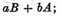the moment of the generated content ABC will be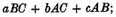and the moments of the generated powers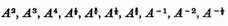will be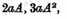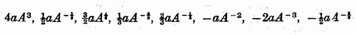respectively; and, in general, that the moment of any power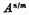will be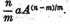Also, that the moment of the genitum A2B will be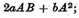the moment of the generated quantity A3B4C2 will be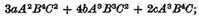and the moment of the generated quantity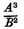or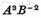will be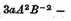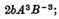and so on. The Lemma is thus demonstrated.

Case 1. Any rectangle, as AB, augmented by a continual flux, when, as yet, there wanted of the sides A and B half their moments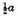and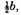was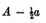into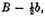or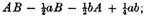but as soon as the sides A and B are augmented by the other half-moments, the rectangle becomes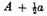into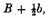or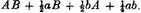From this rectangle subtract the former rectangle, and there will remain the excess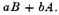Therefore with the whole increments a and b of the sides, the increment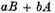of the rectangle is generated. Q.E.D.

Case 2. Suppose AB always equal to G, and then the moment of the content ABC or GC (by Case 1) will be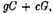that is (putting AB and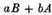for G and g),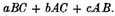And the reasoning is the same for contents under ever so many sides. Q.E.D.

Case 3. Suppose the sides A, B, and C, to be always equal among themselves; and the moment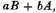of A2, that is, of the rectangle AB, will be 2aA; and the moment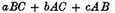of A3, that is, of the content ABC, will be 3aA2. And by the same reasoning the moment of any power An is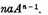Q.E.D.

Case 4. Therefore since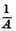into A is 1, the moment of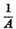multiplied by A, together with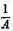multiplied by a, will be the moment of 1, that is, nothing. Therefore the moment of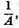or of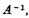is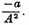And generally since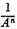into An is 1, the moment of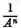multiplied by An together with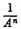into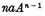will be nothing. And, therefore, the moment of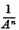or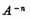wi11 be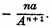Q.E.D.

Case 5. And since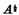into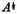is A, the moment of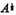multiplied by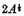will be a (by Case 3); and, therefore, the moment of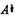will be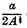or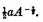And generally, putting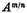equal to B, then Am will be equal to Bn, and therefore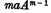equal to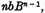and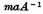equal to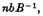or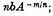and therefore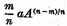is equal to b, that is, equal to the moment of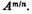Q.E.D.

Case 6. Therefore the moment of any genitum AmBn is the moment of Am multiplied by Bn, together with the moment of Bn multiplied by Am, that is,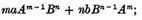and that whether the indices m and n of the powers be whole numbers or fractions, affirmative or negative. And the reasoning is the same for higher powers. Q.E.D.

Corollary I. Hence in quantities continually proportional, if one term is given, the moments of the rest of the terms will be aa the same terms multiplied by the number of intervals between them and the given term. Let A, B, C, D, E, F be continually proportional; then if the term C is given, the moments of the rest of the terms will be among themselves as -2A, -B, D, 2E, 3F.2

Corollary II. And if in four proportionals the two means are given, the moments of the extremes will be as those extremes. The same is to be understood of the sides of any given rectangle.

Corollary III. And if the sum or difference of two squares is given,3 the moments of the sides will be inversely as the sides.

1 A genitum is therefore an expression of one term which is dependent on one variable. The Motte-Cajori translation is genitum, making the term neuter, but in Latin it is quantitas genita, a generated quantity.

2 When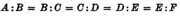and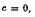then C is constant and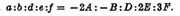3 When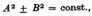then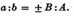Newton, who in Book I has used for his proofs only his theorem of prime and ultimate ratios, in the sections of Book II following his lemma on genita uses occasionally that part of his fluxional calculus which is expressed by the lemma, that is, the formula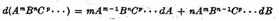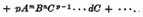Newton’s notation is usually like our own, but occasionally he writes for A2 either Aq, or A quad.

Contents:

### Related Resources

Sir Isaac Newton

Title: A Source Book in Mathematics, 1200-1800

Select an option:

## Email Options

Title: A Source Book in Mathematics, 1200-1800

Select an option: